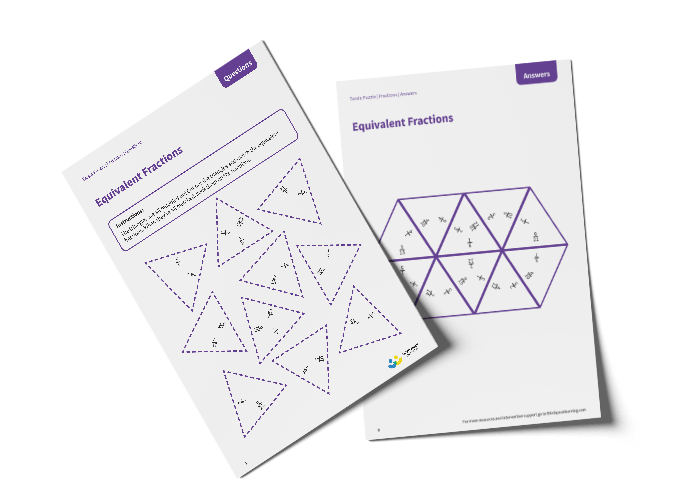# Tarsia Puzzle Equivalent Fractions (Year 3)

The triangles in the tarsia puzzle have all been mixed up. In this equivalent fractions tarsia puzzle, pupils will need to match the two equivalent fractions to create one large hexagon.

This Year 3 equivalent fractions tarsia puzzle worksheet contains:

• One question sheet
• One template sheet

Year 3

Fractions